﻿ 基于环比增长的销售统计分析——2019年中青杯全国数学建模竞赛C题

# 基于环比增长的销售统计分析——2019年中青杯全国数学建模竞赛C题Statistical Analysis of Sales Based on Sequential Growth—2019 Zhongqing Cup National Mathematical Contest in Modeling C Question

Abstract: Firstly, this paper standardizes the sales data of 2016~2018 years by using the formula of input standardization. According to the statistical analysis, the number of standard containers sold for products A, C and E in different regions at different times is E, A and C in descending order. Influ-enced by online shopping and other factors, MT sales channels are gradually shrinking; the model of quarter-on-quarter growth rate prediction is established again, and the range of quar-ter-on-quarter growth rate of six regions in the second quarter of 2019 is predicted by the method of range formula. Finally, under the assumption of constant cost, the rationality of price adjustment is analyzed statistically.

1. 问题背景

2. 模型建立及求解

2.1. 针对问题一的模型建立及求解

$B=\frac{R×X}{24}$

R：销售数据的原始入数；X：销售箱数；

Table 1. The sales volume of products is classified into standardized statistics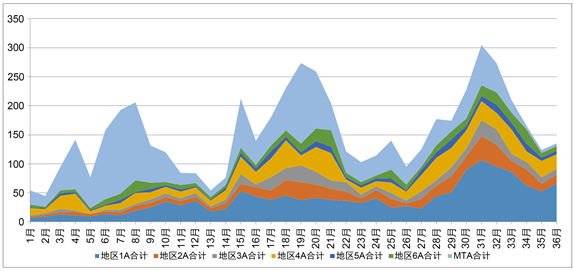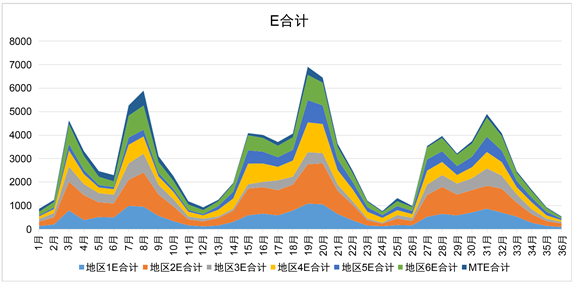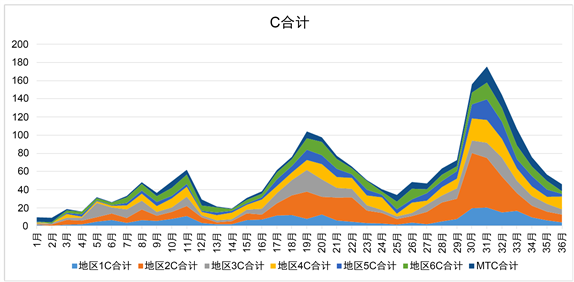Figure 1. Three kinds of products each month summary change trend

2.2. 针对问题二的模型建立及求解

${K}_{i,2}=\frac{{J}_{i,2}-{J}_{i,1}}{{J}_{i,1}}$

${K}_{2019,2}={K}_{2018,2}±C$

$C=\underset{i=2016,2017}{Max}|{K}_{i,2}-{K}_{i+1,2}|$

${J}_{2019,2}=\left(1+{K}_{2019,2}\right){J}_{2019,1}$Table 2. Q2 sequential growth rate and range of total salesTable 3. The sequential growth rate and total sales range of different products in 2019

2.3. 针对问题三的模型建立及求解

2.3.1. 对公司调价决策优缺点的评价

${L}_{ki}=\left({b}_{ki}-{a}_{i}\right){\sum }_{t=1}^{6}{\sum }_{j=1}^{12}{m}_{ijt}$

${b}_{ki}$ ：表示三阶价格； ${a}_{i}$ ：表示成本； ${m}_{ijt}$ ：表示箱数；

$Z{L}_{i}={L}_{2018,i}-{L}_{2017,i}$Table 4. The profit margin of the adjusted product

2.3.2. 对销售决策提出合理方案

1) 从产品类别与销售季度的关系角度：

${K}_{i,j+1}=\frac{{J}_{i,j+1}-J{}_{i,j}}{{J}_{i,j}},$

${K}_{i,j}$ ：第i年第j季度的环比增长率；

$i=2016,2017,2018,j=1,2,3$Table 5. Forecast and analyze sales data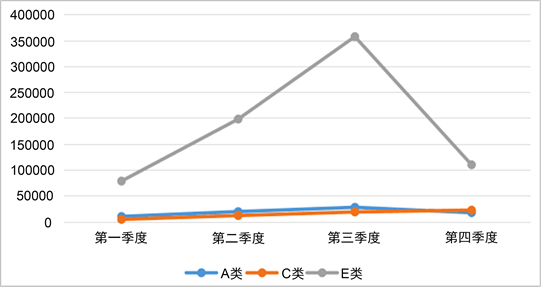Figure2. Sales of the three categories in 2019

2) 从销售地区与销售季度的关系角度：Table 6. Statistical analysis of data from different regions and MT channels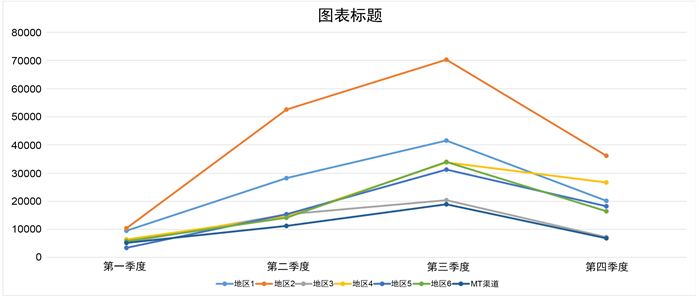Figure 3. Total sales of the six regions and MT channels in different quarters

① 从产品类别的角度：该公司应增加E类产品在2019年第二和第三季度的生产量，

② 从销售地区的角度：该公司应加大对地区2的市场供应量，且高于其他各地区和

MT渠道，在2019年后三季度MT渠道的供应量为最低。在2019年第二季度和第三季度对各地区和MT渠道的供应量都要增加，但在第四季度各地区和MT渠道的供应量均要减少。

3. 总结

 姜启源, 谢金星, 叶俊. 数学模型[M]. 第三版. 北京: 高等教育出版社, 2005.

 李爱强. 统计学基础[M]. 北京: 北京邮电大学出版社, 2016.

 哈金才, 秦传东, 范亚静. 概率论与数理统计[M]. 长春: 吉林大学出版社, 2018.

 伍昊. 你早该这么玩Excel [M]. 北京: 北京大学出版社, 2011.

 熊高强, 陈志雄. 市场营销学[M]. 沈阳: 东北大学出版社, 2018.

Top